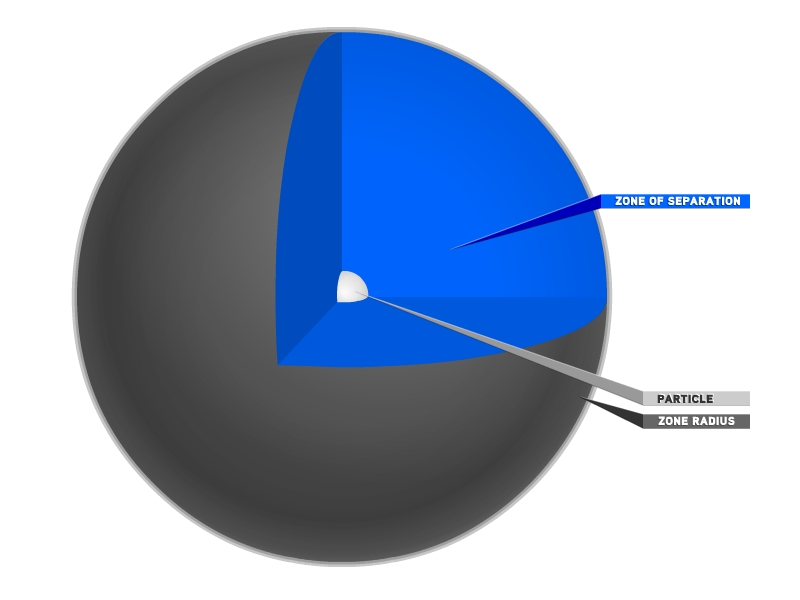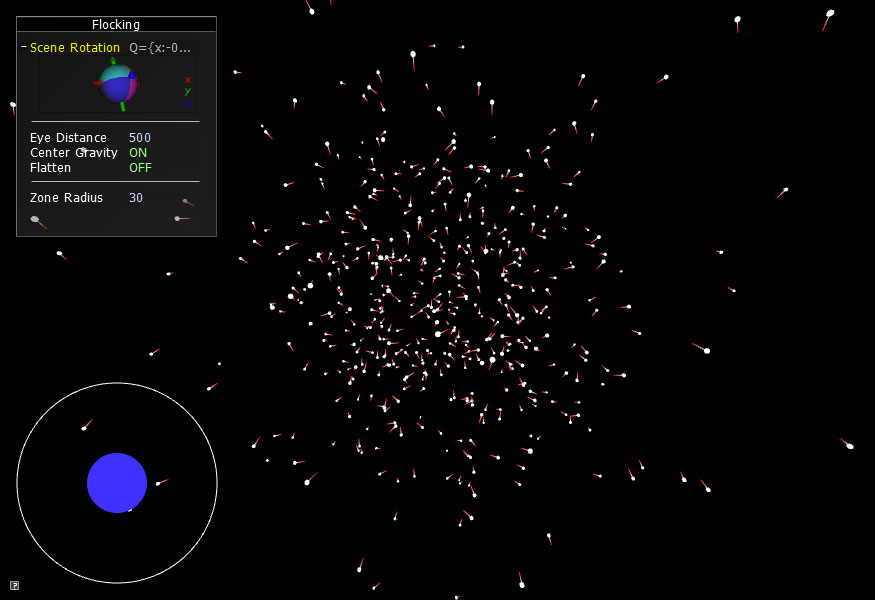# RULE 1: SEPARATION (Avoid crowding)The first rule of flocking is that objects want their own space. They will do what they can to avoid overcrowding. If two objects are moving towards each other, the closer they get, the more they are compelled to adjust their velocity so that they begin to move away from each other. Since we are building on the work of Reynolds, we are going to use his naming conventions for the forces that act on flocking objections. The repulsion force from Hello, Cinder: Section 1, Chapter 5 will now be called a separation force.

```void ParticleController::applyForce( float zoneRadiusSqrd )
{
for( list::iterator p1 = mParticles.begin(); p1 != mParticles.end(); ++p1 ) {

list::iterator p2 = p1;
for( ++p2; p2 != mParticles.end(); ++p2 ) {
Vec3f dir = p1->mPos - p2->mPos;
float distSqrd = dir.lengthSquared();

if( distSqrd <= zoneRadiusSqrd ) { // SEPARATION
float F = ( zoneRadiusSqrd/distSqrd - 1.0f ) * 0.01f;
dir = dir.normalized() * F;
p1->mAcc += dir;
p2->mAcc -= dir;
}
}
}
}```

We use two nested list iterators to compare each Particle with every other Particle. As you can imagine, this slows down dramatically as the number of Particles increases. For the purpose of this tutorial, we are going to use 500 Particles. Every one of these 500 Particles will compare its position to the position of the other 499 Particles. The distance between these objects is noted and if the distance is less than our defined threshold, then apply the separation force.

Finding the square-root of a number is computationally heavy, and it's necessary to find the distance between two points. But there's a simple optimization we can make here because we don't actually need the true distance between the two particles. We just want to see if their distance is less than the zoneRadius. Consider this: if A < B then A^2 < B^2. So if distance < zoneRadius, then distance^2 < zoneRadius ^ 2. And distance^2 is much faster to compute, since it doesn't involve a sqrt() which is notoriously processor intensive. So we will use lengthSquared() instead of length().

To clarify further, to find the length of the vector between two points, we would solve sqrt( x*x + y*y + z*z ). If we want to find out the square of the length of the same vector, we would just need to solve x*x + y*y + z*z. By using lengthSquared(), we are saving ourselves 124,750 sqrt() calls per frame.

The F (force) variable represents the strength of the force acting on the Particles. This variable is definitely up for personal interpretation. For example, if we were simulating the repulsion between two hydrogen atoms, we would know what the force would be because it is something that can be derived mathematically (mostly). However, the force acting between two flocking objects like birds or fish is not something that can yet, if ever, be measured. (When I start messing around with flocking simulations, I spend a great deal of time fine tuning this F number. Really tiny changes can create a stunning number of different behaviors.) So when we say . . .

float F = ( zoneRadiusSqrd/distSqrd - 1.0f ) * 0.01f;

. . . it isn't because we are privy to some cosmic equations or laws. It is all guesswork and a lot of trial and error. In our case, we want the strength of the force to be a fraction of the inverse square of their distance from each other. The 0.01f was chosen simply because 0.1f was way too strong and 0.001f was way too weak. The image below shows an approximation of how the force changes in relation to the distance between the particles.The next line takes the result of our force equation and uses it to scale a normalized vector.

dir = dir.normalized() * F;

Remember, a normalized vector is a vector that has a length of 1.0. Before this equation, dir represents a vector pointing from p1's position to p2's position. Since we are interested in the direction but not the distance, we normalize the vector. Then we multiply this normalized vector by the result of our force equation and we end up with a vector we can use to influence the movement of both Particles.

# RULE 1: SEPARATION (Avoid crowding)

Because we are dealing with a Euler approach to particles (as opposed to the more accurate but more complex Verlet or Runge-Kutta), we need to find a way to deal with the eventuality that two particles will end up being too close to each other and therefore be subject to massive repulsive forces which would send them flying offscreen. We do this by way of a speed limit. If the particle ever goes faster than our max speed, we find the velocity direction and multiply it by the speed limit and use this new velocity instead. Note that we are comparing the square of the distance in order to save us an undesirable sqrt().

float velLengthSqrd = mVel.lengthSquared();
if( velLengthSqrd > mMaxSpeedSqrd ) {
mVel.normalize();
mVel *= mMaxSpeed;
}...

We can also use this method to prevent our Particles from ever reaching equilibrium. We never really want all the Particles to settle into a position permanently. It might be a desirable effect for other types of simulation, but for ours we always want our Particles to be in motion. Therefore, we impose a minimum speed limit as well.

... else if( velLengthSqrd < mMinSpeedSqrd ) {
mVel.normalize();
mVel *= mMinSpeed;
}

If you run the app, you will see something like this:Try adjusting the size of the zone radius to see how it affects the behavior of the Particles. A really small zone leads to the global gravitational force taking over and the Particles congregate near the center of the screen. But as you increase the zone radius, the Particles begin to spread out and the effect of the repulsion becomes apparent.

That concludes Chapter 2. Next, in Flocking Chapter 3, we will be adding our second rule of flocking: cohesion.